International Journal of Applied Mathematics and Theoretical Physics
Volume 2, Issue 4, October 2016, Pages: 46-51

Generalized Equations for the Collinear Doppler Effect

Steven D. Deines

Donatech Technologies Inc., Fairfield, Iowa, USA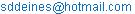Steven D. Deines. Generalized Equations for the Collinear Doppler Effect. International Journal of Applied Mathematics and Theoretical Physics. Vol. 2, No. 4, 2016, pp. 46-51. doi: 10.11648/j.ijamtp.20160204.14

Received: August 6, 2016; Accepted: September 23, 2016; Published: October 19, 2016

Abstract: Some physics textbooks state that the equation for the collinear Doppler effect applies only to a reference frame fixed on the medium, while several textbooks ignore this limitation. The wavelength recorded by a moving observer can be transformed by the textbook Doppler equation in terms of only the source’s frequency and velocity, which demonstrates the textbook equation is inaccurate with a stationary source. The equation for the Doppler effect in textbooks approximates the observer’s frequency, even when the observer’s velocity is much less than the propagation velocity through the medium. The generalized Doppler equations for an observer are derived using infinite series for the moving observer in any inertial frame. The inaccuracy of the textbook equation is due to the false assumption that the observed wavelength in the observer’s frame is the same transmitted wavelength in the frame of the medium. It is also shown for sound that a moving source and moving observer with identical velocities through still air is the equivalent of having a stationary source and stationary observer with a wind of opposite velocity. This particular example also demonstrates that moving interferometers preserve wavelengths. These newly derived Doppler equations for the observer will add more precision with wave phenomena.

Keywords: Doppler Effect, Sound Speed, Waves

Contents

1. Introduction

When a wave source is moving relative to an observer, or an observer is moving relative to the source, the transmitted frequency detected by the observer is different, compared to the propagated frequency when the medium, source, and observer are stationary. This effect is very noticeable when a locomotive whistle sounds as the train approaches the listener standing beside the track and the train passes by the listener. Movement by the source or the observer causes frequency changes, which is known as the Doppler shift. Doppler  was the first to discuss this effect in starlight, but he did not fully derive it . Buys Ballot in 1845 probably was the first to verify the frequency shift via sound in still air by employing listeners who had perfect pitch to record the frequency shift of a steady trumpet sound on a moving train .

Several American physics textbooks fail to identify the inertial frame in which the velocity of the wave phenomena is measured [4-18]. Many physics textbooks do state that the sound velocity is measured relative to the air [19-23,30,32], but fewer textbooks further explain that the source’s and observer’s velocities are also relative to that medium [2,26,28,30,32]. None give the general Doppler equation when the source’s, observer’s or medium’s velocities are measured relative to frames other than the medium. Fowler and Meyer  did qualify that the basic Doppler equation must be modified greatly when the velocity of either the source or observer is not small relative to the propagation velocity. Some textbooks assume stationary air for simplicity [2,7,11,27,29,31].

2. Textbook Derivation of the Equation for the Doppler Effect

Assuming the air or medium is stationary, the textbook derivation is done in two steps: one for the source and the other for the observer. If V is the wave’s velocity through the medium and the source has a velocity, Vs, along the +X axis (e.g. reader’s right), each successive wave will be closer to its predecessor than if the source was stationary. The resulting wavelength will be shorter even though the source’s frequency, fs, remains unchanged.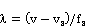(1)

Equation (1) is correct as the propagation is independent of the source’s velocity once the wave is transmitted in the medium and on its way to the observer. If the observer has a positive velocity, VO, on the +X axis, then the observer supposedly collects all the waves in the distance over a unit time interval 1(V – VO) where λ fO = (V – VO) and V > VO. Combine this with (1) to get the observer’s frequency, fO, as: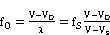(2)

Some references [9,10,20,24] made the statement without proof that if the medium is moving, the wave speed is replaced in (2) for sound by V’ = V + Uw where Uw is the speed of the wind (i.e. medium’s external speed) and V is the speed of sound in still air (i.e. propagation speed within the medium). A wind will move more or fewer nodes past a moving observer per unit time, but (2) predicts no change in frequency if both the source and observer move identically in a windy situation. Consider another case. Insert (2) for fO in the following equation: λO = (V-VO)/fO = (V-Vs)/fs. This implies incorrectly that any observer’s recorded wavelength is only dependent on the source’s velocity and frequency. Equation (2) is inexact when the source is stationary, because any observer’s velocity receding from the source will lengthen the wavelength as shown in Figure 2 of this paper or vice versa in Figure 3.

Equation (2) is the published Doppler equation for the stationary medium with the positive velocities VO and VS parallel with the +X axis. However, each wave overtakes the moving observer at different locations. If the moving observer recedes from the source, the time it takes for two consecutive waves to intercept the observer is Dt for the effective period, but if the observer approaches the source, the period for those same intercepting waves is shorter as dt. The relative velocity of the receding observer is V – VO, while the approaching observer’s relative velocity is V + VO. Equation (2) erroneously assumes the transmitted wave travels the same displacement V x t, regardless which direction the observer is moving. The transmitted wavelength in the medium is not the observed wavelength in the moving observer’s frame. A wavelength is not a rigid interval such as centimeter or inch markings on a solid ruler. Wavelengths are intervals between the reception times of consecutive nodes or antinodes based on the velocity of the wave. This paper’s derivation compensates for the observed wavelength and frequency in the observer’s frame (i.e. V δt < V Δt).

3. Wave Model with Moving Source and Observer

Waves are treated the same here, whether transverse (taut musical strings demonstrating mechanical perpendicular displacements or light via electromagnetic waves) or longitudinal (sound via compressed material or tense springs illustrating mechanical condensation and rarefaction). The chosen model uses sound waves assuming the atmosphere is of uniform density in this derivation, so that a fixed standard temperature and pressure throughout the entire region of horizontal propagation encompasses both the sound source and the observer. To start, assume no wind. The observer is initially displaced one wavelength from the source along the +X axis. The source broadcasts a label from "ZERO" to "NINE" on each antinode upon ten consecutive carrier wavelengths. This assigns a different tag to each antinode in the following diagrams. Both observer and source are constrained along the X axis.

Any perpendicular movement from that straight baseline would create added Doppler effects solved by trigonometry. Most Doppler effects of interest have sufficient displacements between the source and observer, so that collinear movements relegated to the X axis produce virtually all practical frequency shifts. Thus, this derivation will only consider collinear propagation and movement.

In Figure 1, the observer is one wavelength away from the source. The stationary source broadcasts the next incremented wave, while the fixed observer receives the previous wavelength and its message, such as receiving "ZERO" when the source broadcasts "ONE". The transmitted wavelength between the antinodes is shown as λair = vair x Δt where Δt represents exactly the period between consecutive antinodes.Figure 1. Sound Transmissions with Stationary Source and Observer.

Consider the effective wavelength when the observer recedes at a constant speed from the source. At the instant that the observer receives "ZERO" and the source transmits "ONE", the observer instantly recedes from the source at a constant velocity V0. The signal "ONE" arrives at the observer’s former location where it still pursues the observer, and it eventually overtakes the observer as illustrated by the gray "ONE" signal. Even though the transmitted wavelength remains unchanged in the medium, the time of reception exceeds the period between cycles in the medium. In the observer’s frame, the effective wavelength is longer, because "ONE" had to travel further to reach the observer (i.e. the time between "ZERO" and "ONE" is longer). Analytically, the effective wavelength is λeff = λair + Δλo, because the relative velocity between the source and observer is slower as Vrel = V – VO.Figure 2. Transmission of Moving Observer Away From the Source.

In Figure 2, the observer moves right at uniform velocity VO. When the wave travels from the source over the time λ/Vair, the observer is out of reach by being ξ(1) distance further. When that wave travels the extra distance ξ(1), the observer has moved a further distance ξ (2) over the same time interval of ξ(1)/Vair. Over n repetitions of this, the observer has moved a distance of λ + ξ(1) + ξ(2) + … + ξ(n) = λO where ξ(1) = VO × (λ/V) and ξ(i+1) = VO × ξ(i)/V where VVair. Substitute the individual terms with VO < V, and the series is: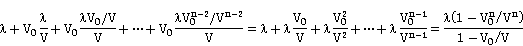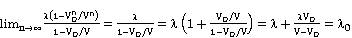(3)

This is the observer’s effective wavelength, λO, transmitted through the air as λ = λair. Consider the situation with a moving observer approaching the source.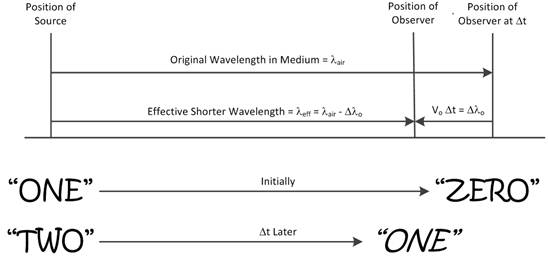Figure 3. Transmission of Moving Observer Towards the Source.

In Figure 3, the observer moves left at uniform velocity VO, at the instant "ZERO" was received, creating a relative velocity Vrel = V + VO. The wave travels a shorter distance than λair as the observer moves toward it. One removes increments of distance ξ(i) instead of adding. To begin, the transmission time is less than λ/V as the observer moved ξ(1) = V0 × λ/V toward the wave by being ξ(1) distance closer. Continue the argument to get the infinite series.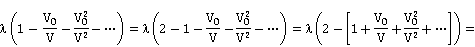(4)

Equations (3) and (4) describe the observed wavelength λO with the transmitted wavelength λ when the observer is moving at a constant speed VO. If the observer is stationary, λ = λO as expected.

All wavelengths and speeds are scalar quantities for the above equations. Equation (4) is the same form as (3) if one considers the observer’s speed as approaching or receding from the source. The moving source has a positive speed if following the propagated waves. The moving observer has a positive speed if receding from the propagated wave. This allows for the Doppler shift to occur when either the source or observer passes the other. Although V was originally the speed of sound in still air as V=Vair, it can be generalized as V′ = Vair + Vwind. Vair is the sound speed relative to the medium and is omnidirectional. The sign for the speed of the wind, Vwind, depends on the direction of the propagated wave within each pair of parentheses. The speed of the observer is positive if receding from the propagated wave, but negative if approaching the wave. For a moving source through the medium, λS = λ = (V′ – VS)/fs where VS is positive if the source is following the propagation of sound or is negative if receding form the propagated sound. The source’s speed modifies λ in (3) and (4). When the source and observer move at different speeds, the general equation for the observed wavelength is: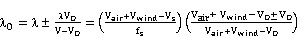(5)

The reference frame’s origin for (5) is located at the onset of sound broadcasts at T0 where the source is initially located. The observer is located on the +X axis. Sound is broadcast as an expanding sphere where the radius increases as |V(T-To)|. If the observer is later located on the opposite side of both the source (Xo – Xs < 0) and the sphere of sound, then the propagated sound will have a negative velocity V. The sign ± becomes positive for a receding observer or negative for an approaching observer relative to the sound source. For the observer’s frequency, use V = fO λO = Vair + Vwind – V0 as: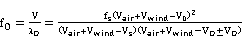(6)

Consider the special situation when both the source and observer move parallel at the same velocity. The source shortens the original wavelength as it moves right, but the observer’s motion effectively elongates the observed wavelength. In Figure 4, the vectors indicate that the wavelength displacement between "ONE" and "ZERO" should be the same between "TWO" and "ONE".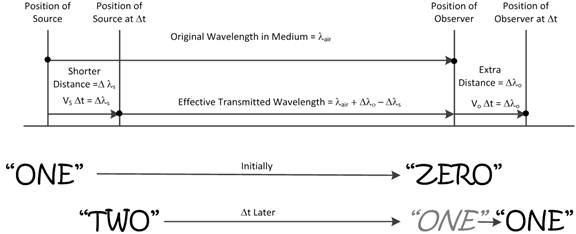Figure 4. Transmission of Moving Source and Observer with Identical Positive Velocities.

Similarly, when both the source and observer move antiparallel to the left with the same velocity, the source creates a longer wavelength while the observer effectively contracts the observed wavelength. In the reference frame of the observer, the vectors demonstrate that the observed wavelength between the anodes "ONE" and "ZERO" is the same between "TWO" and "ONE".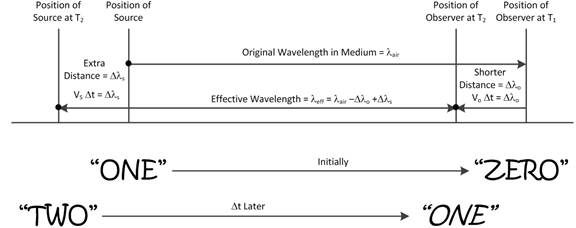Figure 5. Transmission of Moving Source and Observer with Identical Negative Velocities.

The mathematics will confirm this observation. In Figure 4, the moving source causes the wavelength between successive antinodes to be modified by λ = (Vair –Vs) ΔT with one period of ΔT. From (5), the moving observer measures λO = Vair/fs = λ = λS, because Vs = VO in still air. When the wind is opposite the speed of VS and VO in (5), the result λO = (Vair+Vwind)/fs is the same as Vs = VO = 0. From (6), the observed frequency when both source and observer are stationary is the same frequency as the source’s frequency, regardless of the wind. The wind will increase (or decrease) the frequency from the source, but wind will decrease (or increase) the frequency by the same magnitude relative to the observer, so the source’s frequency is retained if both the source and observer are stationary. However, the movement of the observer will alter the received frequency, regardless of the source’s velocity. Thus, the wavelength for both source and observer having identical velocities in still air is interchangeable for the case of both stationary source and observer with a constant wind moving with the opposite velocity.

The example that the source and observer move with identical velocities demonstrates the wavelength of light should be preserved along the arms of a moving interferometer. Each end of the arm has the same velocity, so the number of observed wavelengths that traverse each arm length remains constant. For the Michelson-Morley interferometer, the perpendicular arms are equal, so the recombination of the split beams guarantees maximum coherent interference. The Kennedy-Thorndike interferometer has unequal arms, so the ratio of the observed wavelengths along each arm remains constant and causes a static destructive interference. The observed interference based on recombining split light beams is static in either case. The precision of the interferometer is limited to the uncertainty of the source’s wavelength, the refractive index of the medium and the resolution of interference patterns. This wave property allows interferometers to be precise measurement tools for physics. The surprising result from Figures 4 and 5 implies that neither the Michelson-Morley nor the Kennedy-Thorndike interferometers can prove or disprove the existence of the hypothetical medium for light transmission, because the same static power intensity is output with any velocity of the interferometers (provided V < c). The detailed analysis of this likelihood is beyond the scope of this paper, but it will be examined thoroughly in a future paper.

The published Doppler equation in a windy scenario would not transform to a similar frequency equation in another inertial frame, such as a grounded frame. As already mentioned, (2) erroneously transforms an observed wavelength to be dependent only on the source’s velocity and source’s frequency. Thus, (2) is an approximation. Measurement errors may be large enough to mask the approximate nature of (2) when measuring a source’s and an observer’s velocity precisely relative to a moving medium. If (Vair + Vwind) (Vair + Vwind – VO) (Vair + Vwind – 2VO) for a moving observer, then (6) reduces to the Doppler equation in the textbooks as (2) whenever VO << Vair + Vwind.

4. Conclusion

The textbook form of Doppler’s equation for frequency between a source and observer is found to be an approximation. The precise derivation analyzes the Doppler effect based on wavelengths as the wave intercepts the moving observer. As expected, the moving source causes the transmitted wavelength and frequency in the medium to vary, but the propagation speed is not affected in the reference frame of the medium (See (1)). The moving observer does alter the observed frequency and relative speed of the wave, which (3) and (4) are derived for the two cases of the receding or the approaching observer relative to the sound. The general cases for observed wavelength and frequency by (5) and (6), respectively, apply when the source and observer move at different velocities. When the observer and source undergo the same constant velocity, the original transmitted wavelength is observed. The effective wave speed and frequency for the observer are different depending on the direction of movement of the medium relative to the observer. The observed wavelength when a medium moves at a constant velocity past the stationary observer and source is equivalent to the case of both source and observer moving at the same velocity through a still medium, but in the opposite direction of the moving medium in the prior case. This example also implies that moving interferometers of various speeds always preserve wavelengths. The revised Doppler equations for the observer are applicable for all inertial frames. The Doppler equation published in physics textbooks is an approximation of the exact formulae. This exact formulation for the observer’s Doppler effect should allow more precision in wave phenomena.

References

1. Doppler, C. A. "Über das farbige Licht der Doppelsterne und einiger anderer Gestirne des Himmels",Proceedings of the Bohemian Society of Sciences,1843.
2. Halliday, D., Resnick, R., Walker J., Fundamentals of Physics, 9th ed., Vol. 1, John Wiley and Sons, New York, 2011, p. 461-464.
3. Pickover, C. A., The Physics Book, Sterling, New York, 2011, p. 200.
4. Serway, R. A., and Faughn, J. S., College Physics, 6th ed., Vol. 1, Brooks/Cole Thomson Learning, 2003, p. 435-437.
5. Giambattista, A., Richardson, B. M., and Richardson, R. C., Physics, McGraw Hill, 2008, p. 433-437.
6. Gamon, G. and Cleveland, J. M., Physics: Foundations and Frontiers, Prentice-Hall International, Inc., 1960, p. 140-141.
7. Serway, R. A., and Jewett Jr., J. W., Physics for Scientists and Engineers, 6th ed., Vol. 1, Brooks/Cole Thomson, 2004, p. 522-527.
8. Knight, R. D., Physics for Scientists and Engineers, 2nd ed., Pearson Addison-Wesley, 2007, p. 623-626.
9. Sears, F. W. and Zemansky, M. W., University Physics, 3rd ed., Addison-Wesley Pub. Co., 1963, p. 521-523.
10. Morgan, J., Introduction to University Physics, Allyn and Bacon Inc., Vol 1, 1963, p. 475-478.
11. Jewett Jr., J. W. and Serway, R. A., Physics for Scientists and Engineers with Modern Physics, 8th ed., Vol. 1, Brooks/Cole Cengage Learning, 2010, p. 501.
12. Benumof, R. Concepts in Physics, Prentice Hall, Inc., 1965, p. 438-440.
13. Arens, A. B., Development of Concepts of Physics, Addison-Wesley, 1965, p. 476-477.
14. Semat, H., Fundamentals of Physics, 3rd ed., Hilt, Rinehart, and Winston, New York, 1960, p. 405-409.
15. Baez, A. V., The New College Physics, W. H. Freeman and Co., San Francisco, 1967, p. 228-229.
16. Fowler, R. G. and Meyer, D. I., Physics for Engineers and Scientists, Allyn and Bacon Company, 2nd Ed., 1962, p. 366-368.
17. Resnick, R. and Halliday, D., Physics, Part I, 1st ed., John wiley & Sons, New York, 1966, p. 512-515.
18. Giancoli, D. C. (2005) Physics, 6th ed., Pearson Prentice Hall, p. 338-341.
19. Ohanian, H. C., Physics, 2nd ed., W.W. Norton & Co., New York, 1989, p. 446-450.
20. Tipler, P. A., Physics for Scientists and Engineers, 4th ed., W. H. Freeman & Co., New York, 1999, p. 463-468.
21. Speyer, E., Six Roads from Newton: Great Discoveries in Physics, Wiley Popular Science, NY, 1994, p. 79.
22. Wolf, J., Barron’s AP Physics B, 4th ed., Barron’s Educational Series, Inc., 2008, p. 327.
23. De Pree, G., Physics, Broadway Books, NY, 2004, p. 78.
24. Weber, R. L., Manning, K. V., and White, M. W., College Physics, 4th ed., McGraw-Hill Book Company, 1965, p. 464-465.
25. Weidner, R. T., and Sells, R. L., Elementary Classical Physics, Vol. 2, Allyn and Bacon, Boston, 1965, p. 1049-1052.
26. Shortley, G. and Williams, D., Elements of Physics, Prentice-Hall Int., 4th ed., 1965, p. 463.
27. Cutnell, J. D. and Johnson, K. W., Physics, 9th ed., John Wiley and Sons, 2012, p. 484-490.
28. Young, H. D. and Freedman, R. A., University Physics, 12th ed., Pearson Addison-Wesley, San Francisco, 2007.
29. Halliday, D., Resnick, R., Krane, K.S., Fundamentals of Physics, 4th ed., John Wiley and Sons, New York, 1992, p. 457-460.
30. Rosen, J. and Gothard, L. Q., Encyclopedia of Physical Science, Infobase Publishing, (2009) p. 155, ISBN 0-8160-7011.
31. Serway, R. A. and Jewett Jr., J. W., Physics for Scientists and Engineers, Cengage Learning, 9th ed. (2014), p. 517-519.
32. Young, H. D., Adams, P. W., and Chastain, R. J., Sears and Zemansky’s College Physics, Pearson, 10th ed., (2016) p. 378-381.

 Contents 1. 2. 3. 4.
Article ToolsAbstractPDF(516K)